﻿ 改进的变压器组优化运行控制策略

# 改进的变压器组优化运行控制策略Improved Control Strategy of Optimization Operation of Transformer Bank

Abstract: Boundary division method is the most commonly used transformer economic analysis method, the key is the critical load value. However, the critical load value is only a point value; the actual load is real-time changes, which will inevitably lead to frequent switching of the transformer. In view of this problem, this paper proposes a combination of critical point division and time division of the transformer economic operation control strategy, both to reduce the transformer loss and reduce the number of transformer switching, to achieve the economic operation of the transformer. Taking two transformers as an example, the advantages and disadvantages of this method are verified by analyzing and comparing the loss and switching times of different switching strategies.

1. 引言

2. 临界点划分法

$\Delta {P}_{ZA}={P}_{OZA}+{\left(\frac{S}{{S}_{N}}\right)}^{2}{P}_{KZA}$ (1)

$\Delta {P}_{ZAB}={P}_{OZAB}+{\left(\frac{S}{2{S}_{N}}\right)}^{2}{P}_{KZAB}$ (2)

${S}_{LZ}^{A~AB}=2{S}_{N}\sqrt{\frac{{P}_{OZB}}{3{P}_{KZA}-{P}_{KZB}}}$ (3)

${S}_{LZ}^{A~B}<{S}_{N}$ 情况下可以直接根据变压器经济运行的临界负载功率将其分为两种方式，即当 $S<{S}_{LZ}^{A~B}$ 时，由变压器A单独供电；当 $S>{S}_{LZ}^{A~B}$ 时，由变压器A和B一起供电。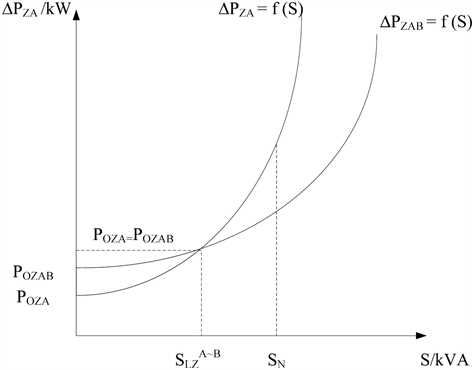Figure 1. The relationship between transformer loss and load

3. 时段划分法

1、若 $\left\{a\left(i+1\right)-a\left(i\right)\right\}>\left({a}_{\mathrm{max}}-{a}_{\mathrm{min}}\right)/24$ ，则规定A的值为1，表示此段时间内负荷处于上升状态。

2、若 $|a\left(i+1\right)-a\left(i\right)|<\left({a}_{\mathrm{max}}-{a}_{\mathrm{min}}\right)/24$ ，则规定A的值为0，表示此段时间内负荷处于不变状态。

3、若 $\left\{a\left(i+1\right)-a\left(i\right)\right\}<\left\{-\left({a}_{\mathrm{max}}-{a}_{\mathrm{min}}\right)/24\right\}$ ，则规定A为值为−1，表示此段时间内负荷处于下降状态。

1、若 $|b\left(i\right)|>0$$|b\left(i+1\right)|=0$ ，则规定 $b\left(i+1\right)$ 的值和 $b\left(i\right)$ 的值相等。

2、若 $|b\left(i\right)-b\left(i-1\right)|+|b\left(i+1\right)-b\left(i\right)|=4$ ，则规定 $b\left(i\right)$ 的值和 $b\left(i-1\right)$$b\left(i+1\right)$ 的值相等。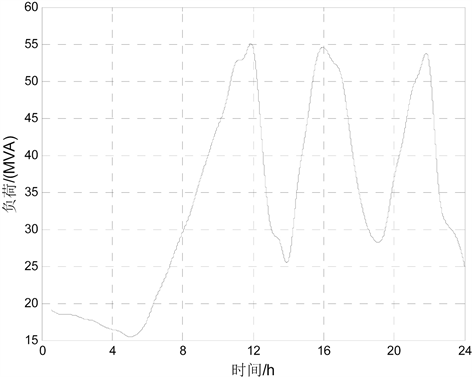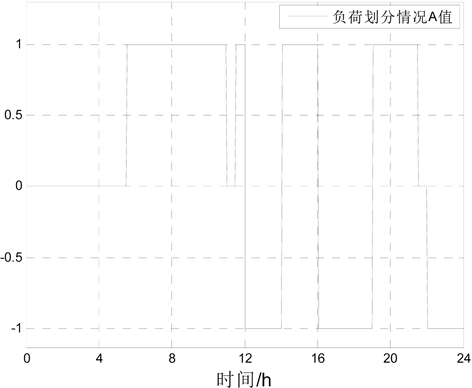Figure 3. The initial division of the load situation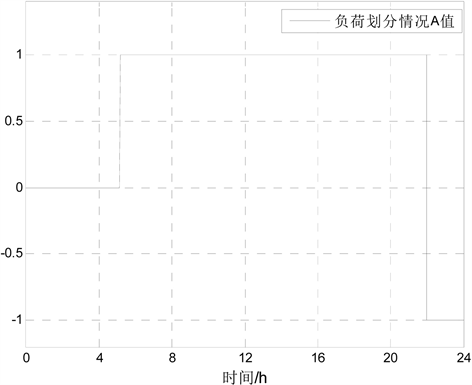Figure 4. The final division of the load

4. 改进的变压器经济运行投切策略分析

4.1. 改进的变压器经济运行理论分析

1、通过对变压器经济运行方式的分析，利用临界点划分法计算公式求出不同运行方式的临界负荷值。

2、进行负荷预测，得到预测日24时段的负荷值，并通过时段划分法对曲线进行简化处理，得到只有一个波峰的矩形曲线。

3、为了改善临界点划分法带来的频繁投切问题，改进的变压器经济运行投切策略将时段划分法与之相结合，这样不只减少了变压器的投切次数，同时也降低了变压器的损耗。具体规则为按照时段划分法处理过的负荷曲线，分析负载值与临界点划分法得出的临界负载值的大小关系，决定变压器的投切时机。在负荷处于上升状态时，实际运行负荷值如果超过变压器并、分列运行的临界负荷值，此时可以投入备用变压器，并且此段时间内不可以退出。在负荷处于下降状态时，实际运行负荷值如果低于变压器并、分列运行的临界负荷值，此时可以退出备用变压器，并且此段时间内不允许再次投入。在负荷保持不变阶段，需要根据实际负荷的发展趋势做出改变。

4.2. 算例分析

${S}_{\delta LP}^{A~B}=\sqrt{\frac{{P}_{OA}-{P}_{OB}}{\frac{{P}_{KB}}{{S}_{NB}^{2}}-\frac{{P}_{KA}}{{S}_{NA}^{2}}}}=398.4157\left( k W \right)$

${S}_{\delta LP}^{A~AB}=\sqrt{\frac{{P}_{OB}}{\frac{{P}_{KA}}{{S}_{NA}^{2}}-{D}_{A}^{2}\frac{{P}_{KA}}{{S}_{NA}^{2}}-{D}_{B}^{2}\frac{{P}_{KB}}{{S}_{NB}^{2}}}}=752.5344\left( k W \right)$

${S}_{\delta LP}^{B~AB}=\sqrt{\frac{{P}_{OA}}{\frac{{P}_{KB}}{{S}_{NB}^{2}}-{D}_{A}^{2}\frac{{P}_{KA}}{{S}_{NA}^{2}}-{D}_{B}^{2}\frac{{P}_{KB}}{{S}_{NB}^{2}}}}=616.6922\left( k W \right)$Table 3. The critical point division method is obtained by the transformer operation mode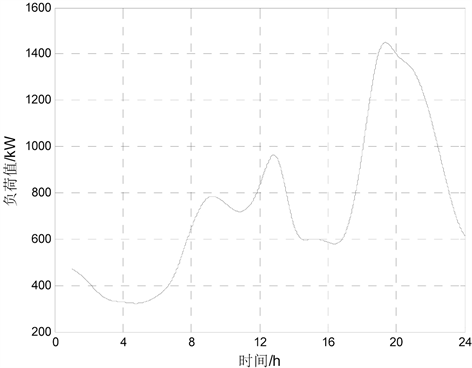Figure 5. Calculation of load forecasting curve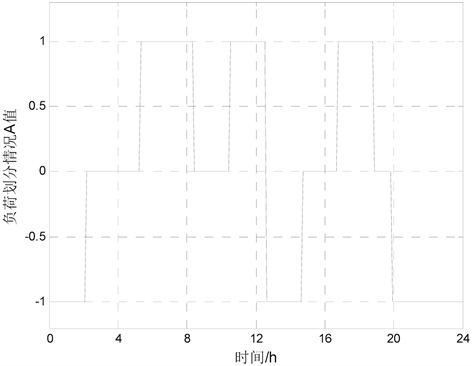Figure 6. Preliminary results of case study load division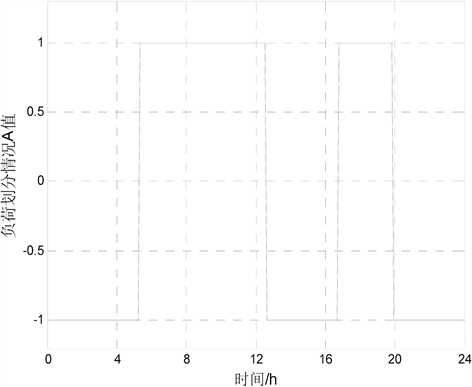Figure 7. Calculate the load division again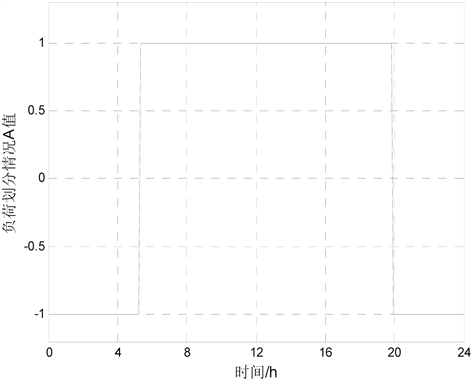Figure 8. Calculate the final result of the loadTable 5. Comparison of switching times in different waysTable 6. Comparison of before and after optimization

5. 结论

 董志恒, 胡国元. 电力变压器经济运行[J]. 电气制造, 2012(11): 32-36.

 秦伟峰. 基于变压器经济运行的10 kV配电网节能降损研究[J]. 中国高新技术企业, 2013(28): 84-85.

 马晓通. 郑州大学配电网变压器经济运行及综合测控系统研究[D]: [硕士学位论文]. 郑州: 郑州大学, 2012.

 许迪. 综合考虑网损及投资运行费用的变压器经济运行区间探讨[J]. 电气开关, 2012, 4(3): 10-13.

 常炳双, 辛健. 配电变压器经济运行模式的探讨[J]. 电网技术, 2007, 31(1): 247-248.

 卢志刚, 李爽, 韩彦玲, 等. 配电网技术经济运行区域的研究及应用[J]. 高电压技术, 2007, 33(6): 156-159.

 潘丹涛. 配电变压器的经济运行[J]. 东北电力技术, 2011, 2(3): 30-32.

 余楚云. 配电系统变压器经济运行方式及其最优投切策略研究工[D]: [硕士学位论文]. 上海: 上海交通大学, 2009.

 周和平, 张艳秋. 现行电价政策与变压器经济运行[J]. 电气时代, 2011(3): 68-70.

 张旭明, 张焰, 汪宇霆, 等. 采用禁忌搜索算法的多时段变压器经济运行方式优化[J]. 电网技术, 2010, 34(7): 109-113.

 张泓泽, 王宝, 郭森. 计及负荷增长的台区配电变压器容量最优选择[J]. 电工技术, 2012(4): 22-24.

 Samet, H., Ghanbari, T. and Ghaisari, J. (2015) Maximum Performance of Electric Arc Furnace by Optimal Setting of the Series Reactor and Transformer Taps Using a Nonlinear Model. IEEE Transactions on Power Delivery, 30, 764-772.
https://doi.org/10.1109/TPWRD.2014.2336693

Top